JEE  >  VITEEE PCME Mock Test - 2

# VITEEE PCME Mock Test - 2

Test Description

## 125 Questions MCQ Test VITEEE: Subject Wise and Full Length MOCK Tests | VITEEE PCME Mock Test - 2

VITEEE PCME Mock Test - 2 for JEE 2023 is part of VITEEE: Subject Wise and Full Length MOCK Tests preparation. The VITEEE PCME Mock Test - 2 questions and answers have been prepared according to the JEE exam syllabus.The VITEEE PCME Mock Test - 2 MCQs are made for JEE 2023 Exam. Find important definitions, questions, notes, meanings, examples, exercises, MCQs and online tests for VITEEE PCME Mock Test - 2 below.
Solutions of VITEEE PCME Mock Test - 2 questions in English are available as part of our VITEEE: Subject Wise and Full Length MOCK Tests for JEE & VITEEE PCME Mock Test - 2 solutions in Hindi for VITEEE: Subject Wise and Full Length MOCK Tests course. Download more important topics, notes, lectures and mock test series for JEE Exam by signing up for free. Attempt VITEEE PCME Mock Test - 2 | 125 questions in 150 minutes | Mock test for JEE preparation | Free important questions MCQ to study VITEEE: Subject Wise and Full Length MOCK Tests for JEE Exam | Download free PDF with solutions
 1 Crore+ students have signed up on EduRev. Have you?
VITEEE PCME Mock Test - 2 - Question 1

### The maximum value of sinx(1 + cos x) will be at

VITEEE PCME Mock Test - 2 - Question 2

### The area, in square unit, bounded by the curves y = x3, y = x2 and the ordinates x = 1, x = 2 is

VITEEE PCME Mock Test - 2 - Question 3

### If z₁ and z₂ are two non-zero complex numbers such that |z₁ + z₂| = |z₁| + |z₂|, the value of arg(z₁) - arg(z₂) is

VITEEE PCME Mock Test - 2 - Question 4

If n is any positive integer ,then the value of
[i(4n+1) - i(4n-1)] /2 equals

VITEEE PCME Mock Test - 2 - Question 5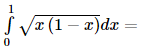VITEEE PCME Mock Test - 2 - Question 6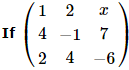is a singular matrix, then x =

VITEEE PCME Mock Test - 2 - Question 7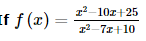for x ≠ 5 and f is continuous at x = 5 then f(5) =

VITEEE PCME Mock Test - 2 - Question 8

If x ≠ 0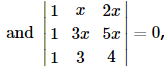, then x =

VITEEE PCME Mock Test - 2 - Question 9

If the function f is defined by f(x)   = x / 1 + | x | then at what points is f differentiable

VITEEE PCME Mock Test - 2 - Question 10

The differential equation for the family of curves x2 + y2 - 2ay = 0, where a is an arbitrary constant is

VITEEE PCME Mock Test - 2 - Question 11

The slope of the tangent at (x,y) to a curve passing thro' a point (2,1) is x2+y2/2xy, then the eqution of the curve is

VITEEE PCME Mock Test - 2 - Question 12

If x(dy/dx) = y(log y - log x + 1), then the solution of the equation is

VITEEE PCME Mock Test - 2 - Question 13

The solution of differential equation (x+2y3) dy/dx = y is

VITEEE PCME Mock Test - 2 - Question 14

The interval in which y=x2ex is increasing with respect to x, is

VITEEE PCME Mock Test - 2 - Question 15

Let f : R → R be defined by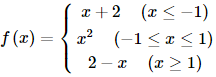Then the value of f(-1.75) + f(0.5) + f(1.5) is

VITEEE PCME Mock Test - 2 - Question 16

∫secx dx=

VITEEE PCME Mock Test - 2 - Question 17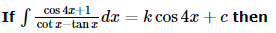VITEEE PCME Mock Test - 2 - Question 18

If cot-1[(cos α)1/2] - tan-1[(cot α)1/2] = x, then sin x =

VITEEE PCME Mock Test - 2 - Question 19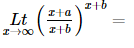VITEEE PCME Mock Test - 2 - Question 20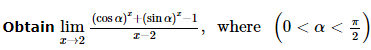VITEEE PCME Mock Test - 2 - Question 21

"If Deb and Sam go to the mall, then it is snowing."
Which statement below is logically equivalent?

Detailed Solution for VITEEE PCME Mock Test - 2 - Question 21

A statement is logically equivalent to its contrapositive.

VITEEE PCME Mock Test - 2 - Question 22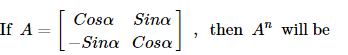VITEEE PCME Mock Test - 2 - Question 23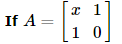and A2  = I then x =

VITEEE PCME Mock Test - 2 - Question 24

Number of numbers greater than 1000 but less than 4000 that can be formed by using the digits 0, 1, 2, 3, 4 when repetition is allowed is

VITEEE PCME Mock Test - 2 - Question 25

Two dice are thrown simultaneously. The probability of getting a pair of 1 is

VITEEE PCME Mock Test - 2 - Question 26

In a Binomial distribution, the probability of getting a success is 1/4 and standard deviation is 3. Then its mean is

VITEEE PCME Mock Test - 2 - Question 27

A and B are two independent events. P( A and B) = 1/6 and the probability that neither of them occur is 1/3 . Find the probability occurrence of A?

Detailed Solution for VITEEE PCME Mock Test - 2 - Question 27

Let P(A) = X and P(B) = Y;
So, P(A’) = 1 - X and P(B’) = 1 - Y
P(A and B) = P(A) x P(B) = XY = 1/6
Probability that neither of them occurs = P(A’) x P(B’) = (1 - X) x (1 - Y) = 1/3
Solving the resulting quadratic equation, we get X = 1/3 or 1/2 that is the probability of occurrence of A

VITEEE PCME Mock Test - 2 - Question 28

The angles of a triangle are in the ratio 3:5:10. Then the ratio of the smallest side to the greatest side is

VITEEE PCME Mock Test - 2 - Question 29

112 + 122+ 132 +....+20is equal to

VITEEE PCME Mock Test - 2 - Question 30

Given n = 10, ∑x = 4, ∑y = 3, ∑x2 = 8, ∑y2 = 9 and ∑xy = 3, then coefficient of correlation is

VITEEE PCME Mock Test - 2 - Question 31

If each observation of a raw data, whose variance is σ2, is multiplied by λ, then the variance of new set is

VITEEE PCME Mock Test - 2 - Question 32
The solution of the equation x+(1/x)=2 is
VITEEE PCME Mock Test - 2 - Question 33

The roots of the equation |x2|+|x|-6=0 are

VITEEE PCME Mock Test - 2 - Question 34

Distance between two parallel planes 2x + y + 2z = 8 and 4x + 2y + 4z + 5 = 0 is

VITEEE PCME Mock Test - 2 - Question 35

The radius of the circle in which the sphere x2 + y2 + z2 + 2x - 2y - 4z - 19 = 0 is cut by the plane x + 2y + 2z + 7 = 0, is

VITEEE PCME Mock Test - 2 - Question 36

The general solution of tan2θ = 3 is

VITEEE PCME Mock Test - 2 - Question 37

The normal to the curve x = a(1+cosθ), y = a sinθ at θ always passes through the fixed point

Detailed Solution for VITEEE PCME Mock Test - 2 - Question 37

Eliminating θ we get (x – a)2 + y2 = a2
Hence normal always pass through (a, 0)

VITEEE PCME Mock Test - 2 - Question 38

The lines 2x - 3y = 5 and 3x - 4y = 7 are diameters of a circle having area as 154 sq.units. Then the equation of the cirlce is

VITEEE PCME Mock Test - 2 - Question 39

The angle between the vectors 2i+3j+k and 2i-j-k is

VITEEE PCME Mock Test - 2 - Question 40

The value of c for which the angle between the vectors cxi-6j+3k, xi+2j+2cxk is obtuse is

VITEEE PCME Mock Test - 2 - Question 41

In a pure inductive circuit or in an A.C. circuit containing inductance only, the current

VITEEE PCME Mock Test - 2 - Question 42

Light rays of wavelengths 6000 Å and of photon intensity 39.6 watts/m2 is incident on a metal surface. If only one percent of photons incident on the surface emit photo electrons, then the number of electrons emitted per second per unit area from the surface will be [ Planck constant = 6.64 x 10-34 J − s ; Velocity of light = 3 x 108  ms-1  ]

VITEEE PCME Mock Test - 2 - Question 43

A capacitor of capacity C₁ is charged by connecting it across a battery of e.m.f. V₀. The battery is then removed and the capacitor is connected in parallel with an uncharged capacitor of capacity C₂. The potential difference across this combination is

VITEEE PCME Mock Test - 2 - Question 44

In connection with chemical effect of current, Faraday constant is equal to

Detailed Solution for VITEEE PCME Mock Test - 2 - Question 44

E ∝ z or E = Fz or F = E/z
Where E = chemical equivalent
z = electrochemical equivalent

VITEEE PCME Mock Test - 2 - Question 45

Intensity of X - rays in coolidge tube is controlled by

VITEEE PCME Mock Test - 2 - Question 46

A laser beam is used for carrying out surgery because it

Detailed Solution for VITEEE PCME Mock Test - 2 - Question 46

As the laser beam is highly monochromatic, directional and coherent, it can be sharply focussed.
Therefore it is used for carrying out surgery

VITEEE PCME Mock Test - 2 - Question 47

Resistance in the two gaps of a meter bridge are 10 ohm and 30 ohm respectively. If the resistances are interchanged the balance point shifts by

VITEEE PCME Mock Test - 2 - Question 48

A resistance R is stretched to four times its length. Its new resistance will be

VITEEE PCME Mock Test - 2 - Question 49

A wire of resistance 12 ohms per meter is bent to form a complete circle of radius 10 cm. The resistance between its two diametrically opposite points, A and B as shown in the Figure, is: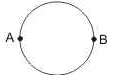VITEEE PCME Mock Test - 2 - Question 50

When a current flows through a conductor its temperature

VITEEE PCME Mock Test - 2 - Question 51

A photon in motion has a mass

VITEEE PCME Mock Test - 2 - Question 52

In a noiseless transformer, an alternating current of 2 ampere is flowing in the primary coil. The no. of turns in the primary and secondary coils are 100 and 20 respectively. The value of the current in secondary coil is

VITEEE PCME Mock Test - 2 - Question 53

In an A.C. circuit, maximum value of voltage is 423 volt. Its effective voltage is

VITEEE PCME Mock Test - 2 - Question 54

Which of the following electromagnetic waves have the smallest wavelength?

VITEEE PCME Mock Test - 2 - Question 55

Choke coil works on the principle of

VITEEE PCME Mock Test - 2 - Question 56

A sphere S1 of radius r1 encloses a total charge Q. If there is another concentric sphere S1 of radius r 2 > r 1 and there be no additional charges between S1 and S2, the ratio of electric flux through S1 and S2 is

VITEEE PCME Mock Test - 2 - Question 57

Two charges -10 C and + 10 C are placed 10cm apart. Potential at the centre of the line joining two charges is

VITEEE PCME Mock Test - 2 - Question 58

Two equal print charges are fixed at x = -a and x = +a on the X axis. Another point charge Q is placed at the origin. The change in electrical potential energy of Q, when it is displaced by a small distance x along the X-axis is approximately proportional to

VITEEE PCME Mock Test - 2 - Question 59

A 5 μ F capacitor is fully charged across a 12V battery. It is then disconnected from the battery and connected to an uncharged capacitor. If the voltage across the capacitor becomes 3V, the capacitance of the uncharged capacitor is

VITEEE PCME Mock Test - 2 - Question 60

The electric potential V is given as a function of distance x (metre) by V = (5x2 + 10x - 9) volt. Value of electric field at x = 1m is

Detailed Solution for VITEEE PCME Mock Test - 2 - Question 60

V = 5x2 + 10x - 9
E = - dv / dx
= - (10x + 10)
= -(10 x 1 + 10)
= - 20 V/m

VITEEE PCME Mock Test - 2 - Question 61

A quatity X is given by ε0 L ΔV/Δt , where ε0 is the permittivity of free space, L is a length, ΔV is potential difference and Δt is the time interval. The dimensional formula for X is the same as that of

Detailed Solution for VITEEE PCME Mock Test - 2 - Question 61

0 L] = [capacitance] = [C]
∴ X = ε0L ( ΔV/Δt ) = CΔV/Δt = ΔQ/Δt = current

VITEEE PCME Mock Test - 2 - Question 62

A charged particle moves through a magnetic field in a direction perpendicular to it. then the

VITEEE PCME Mock Test - 2 - Question 63

The average binding energy of the nucleon is

Detailed Solution for VITEEE PCME Mock Test - 2 - Question 63

The binding energy per nucleon of a nucleus is the binding energy divided by the total number of nucleons. Important features of the graph: Excluding the lighter nuclei, the average binding energy per nucleon is about 8 MeV.

VITEEE PCME Mock Test - 2 - Question 64

A radio active nucleus with mass number 'A' splits into the nuclei whose mass numbers are in the ratio 3:2, the ratio of their radii is

VITEEE PCME Mock Test - 2 - Question 65

The source of stellar energy is

VITEEE PCME Mock Test - 2 - Question 66

Photons of energy of 6 eV are incident on a metal surface whose work function is 4 eV. The minimum kinetic energy of the emitted photoelectrons will be

VITEEE PCME Mock Test - 2 - Question 67

Which of the following pairs have linear relationship between themselves when photoelectrons are emitted from a surface :

VITEEE PCME Mock Test - 2 - Question 68

In the formation of a spectrum of white light by a prism, the deviation of violet colour is

VITEEE PCME Mock Test - 2 - Question 69

The majority charge carriers in p - type semiconductor are

VITEEE PCME Mock Test - 2 - Question 70

Application of a forward bias to a p-n junction

VITEEE PCME Mock Test - 2 - Question 71

A p-n photodiode is fabricated from a semiconductor with band gap of 2.8 eV. Which of the following wavelengths it can detect?

VITEEE PCME Mock Test - 2 - Question 72

The difference in the number of wavelengths, when yellow light propagates through air and vacuum columns of the same thickness, is one. The thickness of the air column is
(Refractive index of air μ a = 1.0003 ; Wavelength of yellow light in vacuum 6000 Å )

Detailed Solution for VITEEE PCME Mock Test - 2 - Question 72

Let λa and λ be the wavelengths of yellow light in air and vacuum respectively and va and c be their respective speeds in air and vacuum.
Since the frequency of light is the same in both media, we have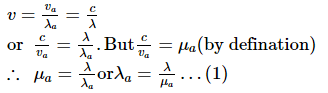Now, if t is the thickness of each columm, then the number of wavelengths in the two media are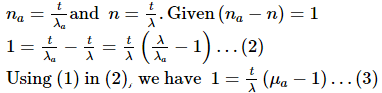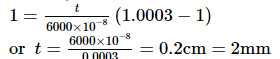VITEEE PCME Mock Test - 2 - Question 73

Which of the following statements is wrong?

VITEEE PCME Mock Test - 2 - Question 74

A semi-conducting device is connected in a series circuit with a battery and a resistance. A current is found to pass through the circuit. If the polarity of the battery is reversed, the current drops to almost zero the device may be

VITEEE PCME Mock Test - 2 - Question 75

In a P-N junction diode

VITEEE PCME Mock Test - 2 - Question 76

Which of the following when added as an impurity into the silicon produces n-type semiconductor?

VITEEE PCME Mock Test - 2 - Question 77

When two coherent monochromatic light beams of intensities I and 4I are superimposed, the ratio between maximum and minimum intensities in the resultant beam is

VITEEE PCME Mock Test - 2 - Question 78

If Young's double slit experiment of light, interference is performed in water, which one of the following is correct?

VITEEE PCME Mock Test - 2 - Question 79

The superposition principle applies to

VITEEE PCME Mock Test - 2 - Question 80

If the ratio of intensities of two waves causing interference be 9:4, then the ratio of maximum and minimum intensities will be

VITEEE PCME Mock Test - 2 - Question 81

3 moles of ethanol react with one mole of phosphorus tribromide to form 3 moles of bromoethane and one mole of X. Which of the following is X?

VITEEE PCME Mock Test - 2 - Question 82

Benzaldehyde can be prepared by the hydrolysis of

VITEEE PCME Mock Test - 2 - Question 83

Which of the following compounds does not react with NaHSO₃?

VITEEE PCME Mock Test - 2 - Question 84

In nucleophilic substitution reactions, the reactivity of carbonyl compounds follows the order

VITEEE PCME Mock Test - 2 - Question 85

The reaction/method that does not give an alkane is

VITEEE PCME Mock Test - 2 - Question 86

The authenticity of an observation, made from an instrument depends upon its

VITEEE PCME Mock Test - 2 - Question 87

Ozonolysis of C₇H₁₄ gave 2-methyl-3-pentanone toluene. The alkene is

VITEEE PCME Mock Test - 2 - Question 88

Assuming full decomposition, the volume of CO₂ released at STP on heating 9.85 g of BaCO₃ (At. mass Ba = 137) will be

VITEEE PCME Mock Test - 2 - Question 89

Leucine amino acids is

VITEEE PCME Mock Test - 2 - Question 90

The hormone which controls the process like burning of fats, proteins and carbohydrates to liberate energy in the body is

Detailed Solution for VITEEE PCME Mock Test - 2 - Question 90

Thyroxine is a hormone secreted by thyroid gland.
This hormone controls various biochemical reactions involving burning of proteins carbohydrates, fats to release energy.

VITEEE PCME Mock Test - 2 - Question 91

Which of the following compounds is responsible for the transmission of heredity characters?

VITEEE PCME Mock Test - 2 - Question 92

Which of the following compounds is formed when benzamide is warmed with bromine and alkali?

VITEEE PCME Mock Test - 2 - Question 93

Formic acid when treated with H₂SO₄ gives

VITEEE PCME Mock Test - 2 - Question 94

Which of the following salt can be produced by the reaction of CO and caustic soda ?

VITEEE PCME Mock Test - 2 - Question 95

A glass bulb is filled with NO₂ gas and immersed in an ice bath at 00C which becomes colourless after some time . This colourless gas will be

VITEEE PCME Mock Test - 2 - Question 96

The rate, at which a substance reacts at constant temperature, depends upon its

VITEEE PCME Mock Test - 2 - Question 97

One mole of an ideal gas at 300 K is expanded isothermally from an initial volume of 1 litre to 10 litres. The ΔE for this process is (R = 2 cal.K-1 mol-1)

Detailed Solution for VITEEE PCME Mock Test - 2 - Question 97

For isothermal reversible expansion of an ideal gas
W = - 2.303n RT log V2/V1
= - 2.303 x 1 x 2 x 300 log 10/1 (log 10 = 1)
= - 2.303 x 2 x 300 = - 1381.8 Cal
[R = 2 Cal mol-1 K-1, T = 300 K]
As ΔE = q + W (1st law of thermodynamics)
For isothermal process q = - W
So ΔE = - W + W = 0
For an isothermal change ΔE = 0
(Since internal energy is a function of temp and in an isothermal process temp remains constant)

VITEEE PCME Mock Test - 2 - Question 98

An endothermic reaction is one in which

VITEEE PCME Mock Test - 2 - Question 99

In which one the following reactions does the heat change represents the heat of formation of water

VITEEE PCME Mock Test - 2 - Question 100

A drug that is antipyretic as well as analgesic is

VITEEE PCME Mock Test - 2 - Question 101

The arrangement of hydrogen atoms around carbon in the methane molecule is

VITEEE PCME Mock Test - 2 - Question 102

Considering H₂O as a weak field ligand, the number of unpaired electrons in [Mn(H₂O)₆]2+ will be (At no.Mn=25)

VITEEE PCME Mock Test - 2 - Question 103

In the presence of acid, the hydrolysis of methyl cyanide gives

VITEEE PCME Mock Test - 2 - Question 104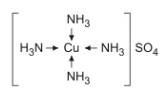The oxidation state of copper in the above complex is

Detailed Solution for VITEEE PCME Mock Test - 2 - Question 104

O.N. of Cu in [Cu (NH3)4] SO4 is +2.

VITEEE PCME Mock Test - 2 - Question 105

Hinsberg reagent is

VITEEE PCME Mock Test - 2 - Question 106

Which of the following shows maximum no. of oxidation states?

VITEEE PCME Mock Test - 2 - Question 107

The specific conductance of a 0.1 N KCl solution at 230C is 0.012 ohm⁻1 cm⁻1. The resistance of cell containing the solution at the same temperature was found to be 55 ohm. The cell constant will be

VITEEE PCME Mock Test - 2 - Question 108

Standard electrode potentials are Fe2+∕Fe (E0 = -0.44) Fe3+∕Fe2+(E0 = 0.77) Fe2+, Fe3+ and Fe blocks are kept together, then

VITEEE PCME Mock Test - 2 - Question 109

Ether is obtained from ethyl alcohol

VITEEE PCME Mock Test - 2 - Question 110

The natural materials from which an element can be extracted economically are called

VITEEE PCME Mock Test - 2 - Question 111

At constant temperature, in a given mass of an ideal gas

VITEEE PCME Mock Test - 2 - Question 112

Which of the following has no tin in its composition?

VITEEE PCME Mock Test - 2 - Question 113

In nitrogen family, the H-M-H bond angle in the hydrides gradually becomes closer to 90o on going from N to Sb. This shows that gradually

VITEEE PCME Mock Test - 2 - Question 114

White phosphorus is

VITEEE PCME Mock Test - 2 - Question 115

SnCl₂ gives a precipitate with a solution of HgCl₂. In this process HgCl₂ is

VITEEE PCME Mock Test - 2 - Question 116

Space lattice of CaF₂ is

VITEEE PCME Mock Test - 2 - Question 117

Types of geometrical isomerism shown at the points x, y and z of the following compound are respectively: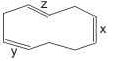VITEEE PCME Mock Test - 2 - Question 118

The CMC is the concentration at which

VITEEE PCME Mock Test - 2 - Question 119

Both acid and base may react with which of the following oxides?

VITEEE PCME Mock Test - 2 - Question 120

Most common oxidation states shown by cerium are

VITEEE PCME Mock Test - 2 - Question 121

Find the antonym of ANTIPATHY

VITEEE PCME Mock Test - 2 - Question 122

Fill in the blank with appropriate word.
He is working under such........conditions that it is difficult to maintain his self-respect.

VITEEE PCME Mock Test - 2 - Question 123

Find the Syonym of ABSOLUTE

VITEEE PCME Mock Test - 2 - Question 124

Improve the sentence by choosing best alternative for capitalised part of the sentence.

It was SHE NOT ME who put forth the attractive proposition.

VITEEE PCME Mock Test - 2 - Question 125

Out of the given alternatives, choose the one which can be substituted for the given capitalised word.

The rebels HELD OUT for about a month.

## VITEEE: Subject Wise and Full Length MOCK Tests

1 videos|3 docs|73 tests
 Use Code STAYHOME200 and get INR 200 additional OFF Use Coupon Code
Information about VITEEE PCME Mock Test - 2 Page
In this test you can find the Exam questions for VITEEE PCME Mock Test - 2 solved & explained in the simplest way possible. Besides giving Questions and answers for VITEEE PCME Mock Test - 2, EduRev gives you an ample number of Online tests for practice

## VITEEE: Subject Wise and Full Length MOCK Tests

1 videos|3 docs|73 tests